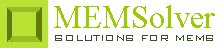Estimate diffusion coefficient of spherical bodies in a solvent

vis        dynamic viscosity in mPa-s
temp     ambient temperature in °C
sel        number denoting the selected result.
Use 2 for diffusion coefficient

Notes

One of the most important parameters required in understanding of the diffusion phenomenon in micro fluidics is the estimation of the diffusion coefficient or diffusion constant of the solute particles in a solvent. The diffusion coefficient determines how fast the solute molecules move a certain distance in the solvent. In this respect an approximate but very useful result can be obtained from Einstein's relation for calculation of diffusion coefficient of Brownian type motion of a spherical body. This is used in the estimation of diffusion coefficient. There could be an error of upto 30%.

The plot shows the diffusion coefficient of spherical bodies ranging from 0.1Å to 50,000Å in radius in water at room temperature (27°C).

Assumptions

-The solution is not highly concentrated.
-The fluid has constant viscosity.
-The viscosity of water is assumed to be 1 mPa-s.